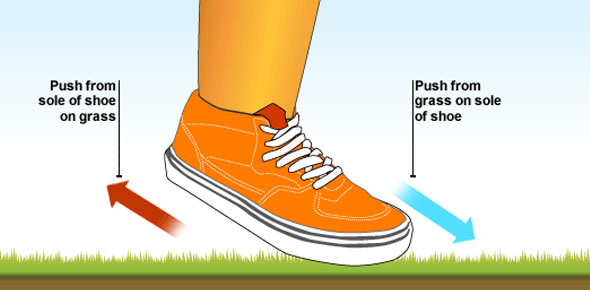# Physics Exam On Motion! MCQ Quiz

20 Questions | Attempts: 569SettingsHow much time will it take a vehicle to travel 50 miles at 60 miles per hour? If you study and take this quiz, you will learn. When something is in motion, it is moving from one place to another. Some things can be in action and remain in the same place as the unfurling of a flag in the wind. The flag itself moves in the wind, but the pole keeps it from flying away. A football being passed from one player to another is in motion from the release to the catch. Find out how much you know by taking the quiz.

• 1.
1. Speed is
• A.

Distance divided by time

• B.

Distance, time and direction

• C.

Both a and b

• 2.
2. Velocity is speed and _________________
• A.

Distance

• B.

Time

• C.

Direction

• 3.
3. When tracking or providing information about a tornado,  which is most important to know?
• A.

Speed

• B.

Time

• C.

Velocity

• 4.
4. Acceleration is the rate at which ______________ changes.
• A.

Velocity

• B.

Time

• C.

Distance

• 5.
5. If a tornado is moving at 50 mph in a NE direction, what is its velocity?
• A.

50 mph N

• B.

50 mph NE

• C.

50 mps NE

• 6.
6. One way you can write speed is
• A.

55 m/h

• B.

55 mph

• C.

Both

• 7.
7. True or False - the surface of any object is rough.
• A.

False

• B.

True

• 8.
8. Kinetic friction is
• A.

Unbalanced or moving

• B.

Balanced or not moving

• C.

Static

• 9.
9. Balanced friction is
• A.

Kinetic

• B.

Static

• C.

Moving

• 10.
• A.

You are sliding on ice and want to stop.

• B.

You are trying to ski on grass.

• C.

You play soccer and you want the ball to roll

• 11.
11. When you roll a ball, you know it will stop, why?
• A.

An unbalanced force will act on it

• B.

Friction will cause the ball to stop

• C.

Two rough surfaces rub together

• D.

All of the above

• 12.
12. Why does a door squeak when you open/close it?
• A.

Not enough friction

• B.

Too much friction

• C.

I like squeaky doors, no problem

• 13.
13. To get grime and food off a pan, you
• A.

Let mom do the hard work

• B.

Add more pressure to the friction

• C.

Use a different sponge

• 14.
14. Gravity pulls matter toward Earth's surface, true or false
• A.

False

• B.

True

• 15.
15. Gravity is the force of attraction between
• A.

Three objects

• B.

Five objects

• C.

Two objects

• 16.
16. As mass increases, gravity's pull increases. This is called
• A.

The law of masses and Earth

• B.

The law of universal gravitation

• C.

The law of friction and balance

• 17.
17. Mass is a measure of
• A.

Gravity's force on an object

• B.

Matter

• C.

Scientific theory

• 18.
18. Weight is a measure of
• A.

Gravity's force on an object

• B.

Matter

• C.

Scientific theory

• 19.
19. The ___________ is the unit of force.
• A.

Kinetic

• B.

Bully

• C.

Newton

• 20.
20. When observing motion, the object that appears to stay in place is called the
• A.

Focal point

• B.

Decimal point

• C.

Reference point

## Related TopicsBack to top
×

Wait!
Here's an interesting quiz for you.